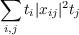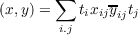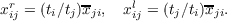#### Vol. 16, No. 2, 1966

 Download this articleFor screen For printingRecent Issues Vol. 325: 1  2 Vol. 324: 1  2 Vol. 323: 1  2 Vol. 322: 1  2 Vol. 321: 1  2 Vol. 320: 1  2 Vol. 319: 1  2 Vol. 318: 1  2Online Archive Volume: Issue:The Journal Subscriptions Editorial Board Officers Contacts Submission Guidelines Submission Form Policies for Authors ISSN: 1945-5844 (e-only) ISSN: 0030-8730 (print) Special Issues Author Index To Appear Other MSP Journals
On two-sided H-algebras

### P. P. Saworotnow

Vol. 16 (1966), No. 2, 365–370
##### Abstract

We call a Banach algebra A, whose norm is a Hilbert space norm, a two-sided H-algebra if for each x A there are elements xl, xr in A such that (xy,z) = (y,xlz) and (yx,z) = (y,zxr) for all y,z A. A two-sided H-algebra is called discrete is each right ideal R such that {xrx R} = {xlx R} contains an idempotent e such that er = el = e. The purpose of this paper is to obtain a structural characterization of those two-sided H-algebras M which consist of complex matrices x = (xiji,j J) (J is any index set) for whichconverges. Here ti is real and 1 ti a for all i J and some real a. The inner product in M isandThen every algebra M is discrete simple and proper (Mx = 0 implies x = 0). Conversely every discrete simple and proper two-sided H-algebra is isomorphic to some algebra M. An incidental result is that the radical of a two-sided H-algebra is the right (left) annihilator of the algebra.

Primary: 46.60
##### Milestones